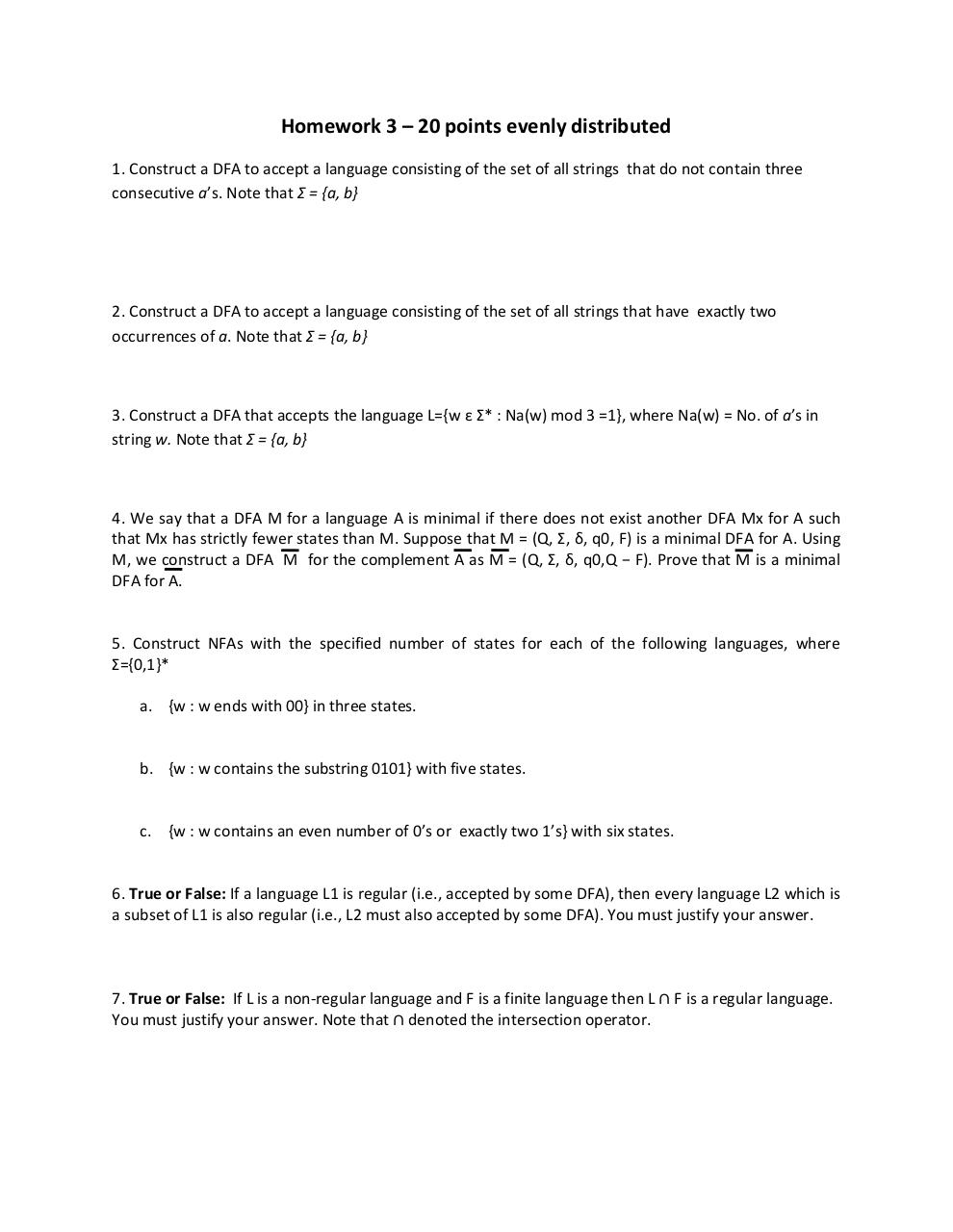# HW3 .pdf

### File information

Original filename: HW3.pdf
Author: chellaps

This PDF 1.5 document has been generated by MicrosoftÂ® Word 2010, and has been sent on pdf-archive.com on 23/09/2015 at 00:57, from IP address 131.151.x.x. The current document download page has been viewed 441 times.
File size: 400 KB (1 page).
Privacy: public file

HW3.pdf (PDF, 400 KB)

### Document preview

Homework 3 – 20 points evenly distributed
1. Construct a DFA to accept a language consisting of the set of all strings that do not contain three
consecutive a’s. Note that Σ = {a, b}

2. Construct a DFA to accept a language consisting of the set of all strings that have exactly two
occurrences of a. Note that Σ = {a, b}

3. Construct a DFA that accepts the language L={w ε Σ* : Na(w) mod 3 =1}, where Na(w) = No. of a’s in
string w. Note that Σ = {a, b}

4. We say that a DFA M for a language A is minimal if there does not exist another DFA Mx for A such
that Mx has strictly fewer states than M. Suppose that M = (Q, Σ, δ, q0, F) is a minimal DFA for A. Using
M, we construct a DFA M for the complement A as M = (Q, Σ, δ, q0,Q − F). Prove that M is a minimal
DFA for A.

5. Construct NFAs with the specified number of states for each of the following languages, where
Σ={0,1}*
a. {w : w ends with 00} in three states.

b. {w : w contains the substring 0101} with five states.

c. {w : w contains an even number of 0’s or exactly two 1’s} with six states.

6. True or False: If a language L1 is regular (i.e., accepted by some DFA), then every language L2 which is
a subset of L1 is also regular (i.e., L2 must also accepted by some DFA). You must justify your answer.

7. True or False: If L is a non-regular language and F is a finite language then L

F is a regular language.#### HTML Code

Copy the following HTML code to share your document on a Website or Blog

#### QR Code### Related keywords